# Dissipative operator

Jump to: navigation, search

A linear operatordefined on a domain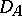which is dense in a Hilbert spacesuch that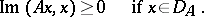This requirement is sometimes replaced by the condition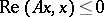if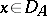, i.e. the dissipativeness ofin this sense is equivalent to that of the operator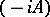.

A dissipative operator is said to be maximal if it has no proper dissipative extensions. A dissipative operator always has a closure, which also is a dissipative operator; in particular, a maximal dissipative operator is a closed operator. Any dissipative operator can be extended to a maximal dissipative operator. For a dissipative operator all pointswith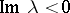belong to the resolvent set, and moreover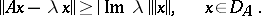A dissipative operator is maximal if and only iffor allwith. An equivalent condition for maximality of a dissipative operator is that it is closed and thatIf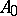is a maximal symmetric operator, then eitheror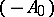is a maximal dissipative operator. Dissipative and, in particular, maximal dissipative extensions may be considered for an arbitrary symmetric operator; their description is equivalent to the description of all maximal dissipative extensions of the conservative operator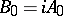:,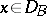.

Dissipative operators are closely connected with contractions (cf. Contraction) and with the so-called accretive operators, i.e. operatorsfor whichis a dissipative operator. In particular, an accretive operatoris maximal if and only if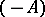is the generating operator (or generator) of a continuous one-parameter contraction semi-groupon. The Cayley transform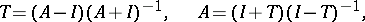whereis a maximal accretive operator andis a contraction not having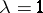as an eigen value, is used to construct the functional calculus and, in particular, the theory of fractional powers of maximal dissipative operators.

In the case of bounded linear operatorsthe definition of a dissipative operator is equivalent to the requirement, whereis the imaginary part of the operator. For a completely-continuous dissipative operatoron a separable Hilbert spacewith nuclear imaginary part, several criteria (i.e. necessary and sufficient conditions) for the completeness of the system of its root vectors are available; for example,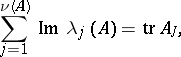whereare all eigen values of the operator,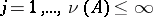, andis the trace of the operator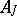(Livshits' criterion);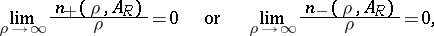where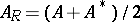is the real part of, and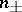is the number of characteristic numbers of the operator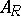in the segmentand(Krein's criterion). The system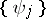of eigen vectors corresponding to different eigen values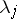,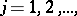of a dissipative operator forms a basis of its closed linear span and is equivalent to an orthonormal basis ifThe concept of a dissipative operator was also introduced for non-linear and even for multi-valued operators. Such an operator on a Hilbert space is called dissipative if for any two of its values the inequality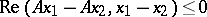holds. This concept also forms the base of the theory of one-parameter non-linear contraction semi-groups and the related differential equations. Another generalization of the concept of a dissipative operator concerns operators acting on a Banach space with a so-called semi-inner product. Another generalization concerns operators acting on a Hilbert space with an indefinite metric.